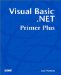# Multidimensional Arrays with More Than Two Dimensions

You can create a multidimensional array that has more than two dimensions. For example, this statement:

` Dim MyCube(10, 5, 3) As Integer `

defines an array named MyCube() with 11 rows, 6 columns , and a depth of 4 integers. This array could be visualized as a cube.

So, how many dimensions can an array have? Visual Basic .NET gives you the ability to define an array with 32 dimensions. However, I'm not as smart as Stephen Hawking and have never used anything with more than 3 dimensions. Indeed, I have tried to think of an example using 4 or more dimensions, and all I have to show for my efforts is a headache .

#### Dynamic Multidimensional Arrays

Earlier in the chapter you learned how to define a dynamic array and use the ReDim statement to redimension an array to a new size. Can you also redimension multidimensional arrays? Suppose you try the following:

` Dim MyArray() As Integer  ReDim MyArray(5, 5) `

If you try to compile a program with these two lines in it, Visual Basic .NET gets upset and tells you ReDim cannot change the number of dimensions of an array . In other words, Visual Basic .NET thinks that the Dim statement is for a one-dimensional array ”information that Visual Basic .NET recorded in its symbol table when it processed the Dim statement. Therefore, the Dim statement must not be conveying the proper information to Visual Basic .NET. How can you tell it to create a multidimensional array in the Dim statement without supplying any index values in the Dim statement?

Remember that when you wanted a one-dimensional dynamic array, you simply left out any value for the index in the Dim statement. Would the same concept apply in a multidimensional dynamic array? Suppose you left out the actual numbers but left in the comma between the two numbers :

` Dim MyArray( , ) As Integer  ReDim MyArray(5, 5) `

These two statements compile without error. Make sure you notice the lonely comma between the parentheses in the Dim statement. The comma tips Visual Basic .NET off to the fact that you want to create a two-dimensional dynamic array.

What if you need a three-dimensional dynamic array? In that case, this allows you to define a three-dimensional dynamic array:

` Dim MyArray( , , ) As Integer  ReDim MyArray(10, 5, 4) `

The rule is simple:

To create an N-dimensional dynamic array, place N “1 commas inside the parentheses in the array's Dim statement.

As you just saw, a two-dimensional dynamic array (that is, N=2) requires one comma inside the parentheses (2 “1=1 comma). A three-dimensional array requires two commas (that is, 3 “1=2). A four-dimensional array requires three commas (that is, 4 “1=3) plus a lot more math skills that I possess.Visual Basic .NET Primer Plus
ISBN: 0672324857
EAN: 2147483647
Year: 2003
Pages: 238
Authors: Jack Purdum

Similar book on Amazon